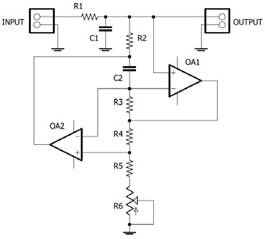# EEO 304-2018 Electronic Instrumentation and Operational Amplifiers

## Homework 5GIC as part of tunable band-pass filter.

Given:

R1 = 1.0 kΩ;

R2 = 1.0 kΩ;

R3 = 1.0 kΩ;

R4 = 1.0 MΩ;

R5 = 1.0 kΩ;

R6 = 10 kΩ;

C1 = 1000 μF;

C2 = 1 μF;

VCC = ± 12 Volt;

OA1, OA2 – 741

• Present 2 equivalent RCL circuits (for R6 = 0Ω and R6 = 10kΩ).
• Find a resonant Frequencies f0min and f0max (for R6 = 0Ω and R6 = 10kΩ).
• Find value of Q for both frequencies.
• Simulate the circuit in the frequency’s range f between 1 and 10,000 Hz. Use Vin=100 mV (RMS);
• Present result in form of Vout / Vin = F(f) for 2 simulations (for R6 = 0Ω and R6 = 10kΩ) on the same logarithmic graph.